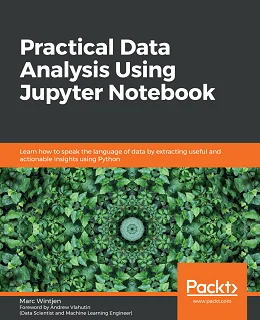# Practical Data Analysis using Jupyter Notebook### eBook Details:

• Paperback: 322 pages
• Publisher: WOW! eBook (June 19, 2020)
• Language: English
• ISBN-10: 1838826033
• ISBN-13: 978-1838826031

### eBook Description:

Practical Data Analysis using Jupyter Notebook: Understand data analysis concepts to make accurate decisions based on data using Python programming and Jupyter Notebook

Data literacy is the ability to read, analyze, work with, and argue using data. Data analysis is the process of cleaning and modeling your data to discover useful information. This book combines these two concepts by sharing proven techniques and hands-on examples so that you can learn how to communicate effectively using data.

After introducing you to the basics of data analysis using Jupyter Notebook and Python, the book will take you through the fundamentals of data. Packed with practical examples, this guide will teach you how to clean, wrangle, analyze, and visualize data to gain useful insights, and you’ll discover how to answer questions using data with easy-to-follow steps.

Later chapters teach you about storytelling with data using charts, such as histograms and scatter plots. As you advance, you’ll understand how to work with unstructured data using natural language processing (NLP) techniques to perform sentiment analysis. All the knowledge you gain will help you discover key patterns and trends in data using real-world examples. In addition to this, you will learn how to handle data of varying complexity to perform efficient data analysis using modern Python libraries.

• Understand the importance of data literacy and how to communicate effectively using data
• Find out how to use Python packages such as NumPy, pandas, Matplotlib, and the Natural Language Toolkit (NLTK) for data analysis
• Wrangle data and create DataFrames using pandas
• Produce charts and data visualizations using time-series datasets
• Discover relationships and how to join data together using SQL
• Use NLP techniques to work with unstructured data to create sentiment analysis models
• Discover patterns in real-world datasets that provide accurate insights

By the end of this Practical Data Analysis using Jupyter Notebook book, you’ll have gained the practical skills you need to analyze data with confidence.